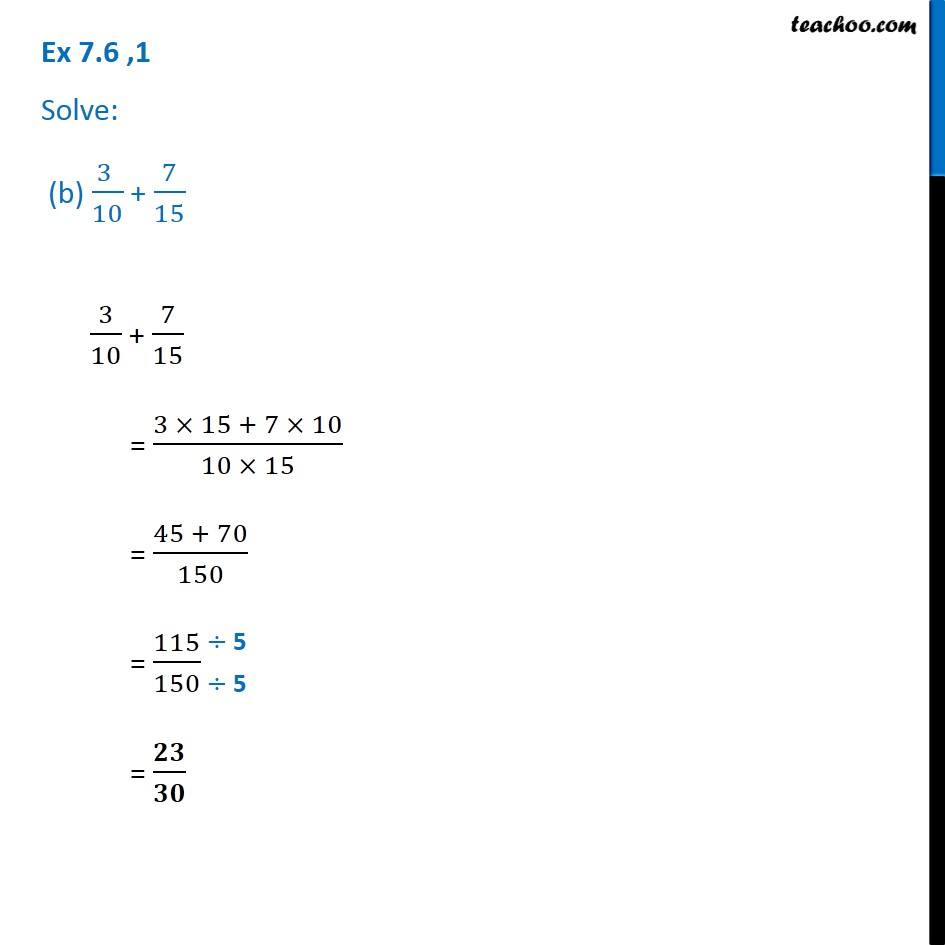1. Chapter 7 Class 6 Fractions
2. Serial order wise
3. Ex 7.6

Transcript

Ex 7.6 ,1 Solve: (b) (3 )/10 + 7/15 3/10 + 7/15 = (3 15 + 7 10)/(10 15) = (45 + 70)/150 = 115/150 = /